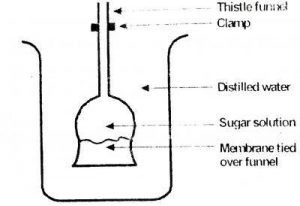## Biology 2018 Past Questions | JAMB

Study the following Biology past questions and answers for JAMBWAEC  NECO and Post-JAMB. Get prepared with official past questions and answers for upcoming examinations.

1. A group of closely related organisms capable of interbreeding to produce the fertile offspring are known as members of a

• A. Kingdom
• B. class
• C. family
• D. species

2. A beaker of pond water containing few specimens of Euglena was placed in a dark room for two weeks. At the end of this period, the specimens of Euglena were still alive because they were

• A. able to carry out holozoic nutrition
• B. able to carry out photosynthesis using carbon dioxide in the pond water
• C. better adapted to life in darkness than to life in light
• D. not overcrowde

3. The cytoplasm of a cell is considered a very important component because it

• A. regulates the amount of energy in the cell
• B. suspends all cell organelles
• C. is the outermost part of the cell
• D. is solely responsible for cell division

4.Use the diagram shown to answer the question. After an hour, the level of water in the thistle funnel will

• A. rise
• B. fall
• C. remain the same
• D. double

5.Use the diagram shown to answer the question. The experiment above is used to demonstrate the process of

• A. transportation
• B. water culture
• C. diffusion
• D. osmosis

6.Use the diagram shown to answer the question. In plant cells, the role of the membrane is played by the

• A. nucleolus
• B. cell wall
• C. cytoplasm
• D. mitochondrion

7. Red blood cells were found to have burst open after being placed in distill for an hour. This phenomenon is known as

• A. plasmolysis
• B. diffusion
• C. haemolysis
• D. wilting

8. The curvature movement of plants in response to the stimulus of water is called

• A. hydrotropism
• B. geotropism
• C. Phototropism
• D. thigmotropism

9.  The overall reaction in glycolysis can be summarised as

• A. C$$_{6}$$H$$_{12}$$O$$_{6}$$ —–> C$$_{3}$$H$$_{4}$$O$$_{3}$$ + 4H + ATP
• B. C$$_{6}$$H$$_{12}$$O$$_{6}$$ —–> 2C$$_{3}$$H$$_{4}$$O$$_{3}$$ + 4H + 2ATP
• C. C$$_{6}$$H$$_{12}$$O$$_{6}$$ —–> 2C$$_{3}$$H$$_{4}$$O$$_{3}$$ + 4H + ADP
• D. C$$_{6}$$H$$_{12}$$O$$_{6}$$ —–> 2C$$_{3}$$H$$_{4}$$O$$_{3}$$ + 4H + 2ADP

10. The longest bone in the body is the

• A. humerous
• B. femur
• C. scapula
• D. tibia
Scroll to Top